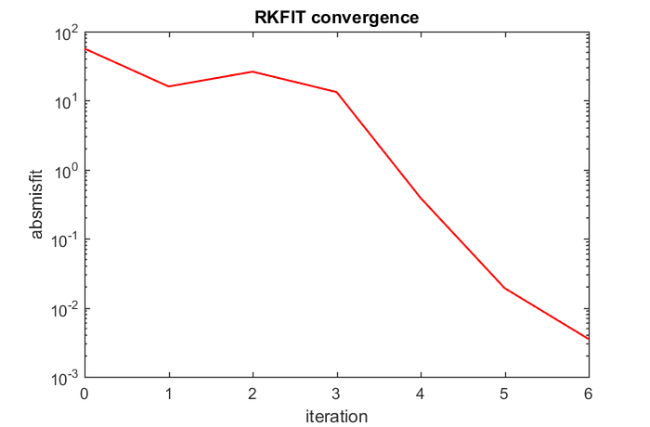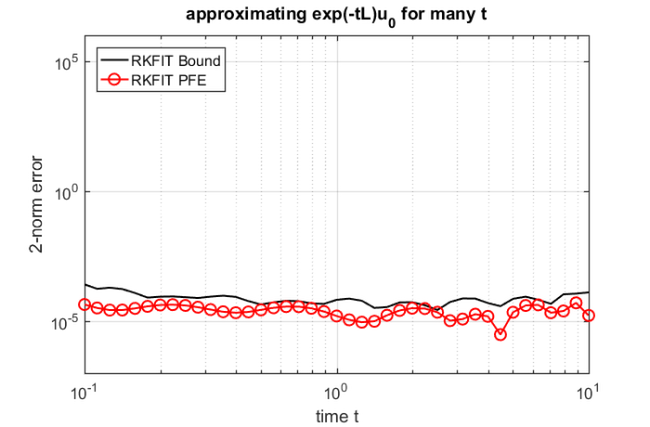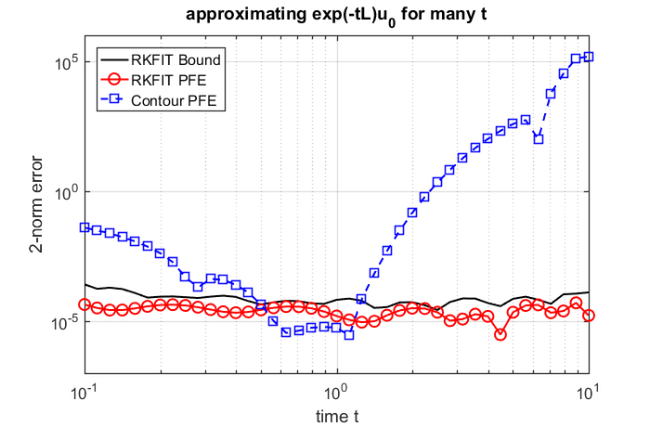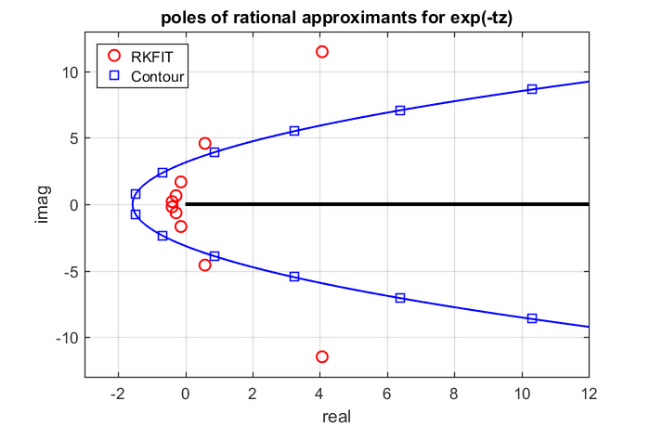# Pole optimization for exponential integration

## Introduction

This example is concered with the computation of a family of common-denominator rational approximants for the two-parameter function $\exp(-tz)$ using RKFIT [2, 3]. This corresponds to the example from [3, Section 6.2]. Let us consider the problem of solving a linear constant-coefficient initial-value problem

$\displaystyle \mathbf{u}'(t) + L \mathbf{u}(t) = \mathbf{0}, \quad \mathbf{u}(0) = \mathbf{u}_0,$

at several time points $t_1,\ldots, t_\ell$. The exact solutions $\mathbf{u}(t_j)$ are given in terms of the matrix exponential as $\mathbf{u}(t_j) = \exp(-t_j L) \mathbf{u}_0$. A popular approach for approximating $\mathbf{u}(t_j)$ is to use rational functions $r^{[j]}$ of the form

$\displaystyle r^{[j]}(z) = \frac{\sigma_1^{[j]}}{\xi_1 - z} + \frac{\sigma_2^{[j]}}{\xi_2 - z} + \cdots + \frac{\sigma_m^{[j]}}{\xi_m - z},$

constructed so that $r^{[j]}(L)\mathbf{u}_0 \approx \mathbf{u}(t_j)$. Note that the poles of $r^{[j]}$ do not depend on $t_j$ and we have

$\displaystyle r^{[j]}(L) \mathbf{u}_0 = \sum_{i=1}^m \sigma_i^{[j]} (\xi_i I - L)^{-1} \mathbf{u}_0,$

the evaluation of which amounts to the solution of $m$ linear systems. Such common-pole approximants have great computational advantage, in particular, in combination with direct solvers (as the LU factorizations of $\xi_i I - L$ can be reused for each $t_j$) and when the linear systems are assigned to parallel processors.

## Surrogate approach

In order to use RKFIT for finding "good" poles $\xi_1,\ldots,\xi_m$ of the rational functions $r^{[j]}$, we propose a surrogate approach similar to that in . Let $A = \mathrm{diag}(\lambda_1,\ldots,\lambda_N)$ be a diagonal matrix whose eigenvalues are a "sufficiently dense" discretization of the positive semiaxis $\lambda\geq 0$. In this example we take $N=500$ logspaced eigenvalues in the interval $[10^{-6},10^6]$. Further, we define $\ell=41$ logspaced time points $t_j$ in the interval $[10^{-1},10^1]$, and the matrices $F^{[j]} = \exp(-t_j A)$. We also define $\mathbf{b}=[1,\ldots,1]^T$ to assign equal weight to each eigenvalue of $A$.

N  = 500;
ee = [0 , logspace(-6, 6, N-1)];
A  = spdiags(ee(:), 0, N, N);
b  = ones(N, 1);
t  = logspace(-1, 1, 41);
for j = 1:length(t)
F{j} = spdiags(exp(-t(j)*ee(:)), 0, N, N);
end


We then run the RKFIT algorithm for finding a family of rational functions $r^{[j]}$ of type $(m-1,m)$ with $m=12$ so that $\| F^{[j]}\mathbf{b} - r^{[j]}(A)\mathbf{b} \|_2$ is minimized for all $j=1,\ldots,\ell$.

m = 12; k = -1;              % type (11, 12)
xi = inf(1,m);               % initial poles at infinity
param.k = k;                 % subdiagonal approximant
param.maxit = 6;             % at most 6 RKFIT iterations
param.tol   = 0;             % exactly 6 iterations
param.real  = 1;             % data is real-valued
[xi, ratfun, misfit, out] = rkfit(F, A, b, xi, param);


## The RKFIT outputs

The first output argument of RKFIT is a vector xi collecting the poles $\xi_1,\ldots,\xi_m$ of the rational Krylov space. The second output ratfun is a cell array each cell of which is a rkfun, a datatype representing a rational function. All rational functions in this cell array share the same denominator with roots $\xi_1,\ldots,\xi_m$. The next output parameter is a vector containing the computed relative misfit after each RKFIT iteration. The relative misfit is defined as (cf. eq. (1.5) in )

$\displaystyle \mathrm{misfit} = \sqrt{\frac{{\sum_{j=1}^\ell \| F^{[j]}\mathbf{b} - r^{[j]}(A)\mathbf{b} \|_F^2}} {{\sum_{j=1}^\ell \| F^{[j]} \mathbf{b} \|_F^2}}}.$

We can easily verify that the last entry of misfit indeed corresponds to this formula:

num = 0; den = 0;
for j = 1:length(ratfun)
num = num + norm(F{j}*b - ratfun{j}(A,b), 'fro')^2;
den = den + norm(F{j}*b, 'fro')^2;
end
disp([misfit(end) sqrt(num/den)])

   3.6468e-05   3.6468e-05


Here is a plot of the misfit vector, giving an idea of the RKFIT convergence:

figure
semilogy(0:6, [out.misfit_initial, misfit]*sqrt(den), 'r-');
xlabel('iteration');
ylabel('absmisfit')
title('RKFIT convergence')## Verifing the accuracy

To evaluate the quality of the common-denominator rational approximants for all $\ell=41$ time points $t_j$, we perform an experiment similar to that in [5, Figure 6.1] by approximating $\mathbf{u}(t_j) = \exp(-t_j L) \mathbf{u}_0$ and comparing the result to MATLAB's expm. Here, $L$ is a ${841\times 841}$ finite-difference discretization of the scaled 2D Laplace operator $-0.02\Delta$ on the domain $[-1,1]^2$ with homogeneous Dirichlet boundary condition, and $\mathbf{u}_0$ corresponds to the discretization of $u_0(x,y) = (1-x^2)(1-y^2)e^x$ on that domain.

% Parts of the following code have been taken from .
J = 30; h = 2/J; s = (-1+h:h:1-h)';  % in [3,5] J = 50 is used
[xx,yy] = meshgrid(s,s);             % 2D grid
x = xx(:); y = yy(:);                % 2D grid stretched to 1D
L = 0.02*gallery('poisson',J-1)/h^2; % 2D Laplacian
v = (1-x.^2).*(1-y.^2).*exp(x);      % initial condition
v = v/norm(v);

for j = 1:length(t)
exac(:,j) = expm(-t(j)*L)*v;
rat = ratfun{j}(L,v);
err_rat(j) = norm(rat - exac(:,j));
bnd(j) = norm(ratfun{j}(A,b) - F{j}*b,inf);
end


We now plot the error $\| \mathbf{u}(t_j) - r^{[j]}(L) \mathbf{u}_0\|_2$ for each time point $t_j$ (curve with red circles), together with the approximate upper error bound $\| \exp(-t_j A)\mathbf{b} - r^{[j]}(A) \mathbf{b} \|_\infty$ (black curve), which can be easily computed by direct evaluation. We find that the error is indeed approximately uniform and smaller than $1.1\times 10^{-4}$ over the time interval $[10^{-1},10^1]$.

figure
loglog(t, bnd, 'k-')
hold on
loglog(t, err_rat, 'r-o')
xlabel('time t'); ylabel('2-norm error')
legend('RKFIT Bound', 'RKFIT PFE', 'Location', 'NorthWest')
title('approximating exp(-tL)u_0 for many t')
grid on
axis([0.1, 10, 1e-7, 1e6])## Conversion to partial fraction form

When evaluating the rational functions $r^{[j]}$ on a parallel computer, it is convenient to have their partial fraction expansions at hand. The rkfun class provides a method called residue for this purpose. This method supports the use of MATLAB's variable precision (VPA) capabilities, or the Advanpix Multiple Precision (MP) toolbox .

For example, here are the residues and poles of the first rational function $r^{}$ corresponding to $\exp(-t_1 A)\mathbf{b}$:

try mp(1); catch e, try mp=@(x)vpa(x); mp(1); catch e, mp=@(x)x;
warning('MP & VPA are unavailable. Using double.'); end, end

[resid, xi, absterm, cnd, pf] = residue(mp(ratfun{1}));
disp(double([resid , xi]))

   1.1058e-01 - 4.2664e-03i  -3.9348e-01 + 1.9680e-01i
1.1058e-01 + 4.2664e-03i  -3.9348e-01 - 1.9680e-01i
1.2375e-01 + 9.7078e-03i  -2.7724e-01 + 6.4704e-01i
1.2375e-01 - 9.7078e-03i  -2.7724e-01 - 6.4704e-01i
2.7683e-01 - 2.6172e-02i  -1.3284e-01 + 1.6788e+00i
2.7683e-01 + 2.6172e-02i  -1.3284e-01 - 1.6788e+00i
5.0072e-01 - 4.3971e-01i   5.8293e-01 + 4.5857e+00i
5.0072e-01 + 4.3971e-01i   5.8293e-01 - 4.5857e+00i
-2.1039e-01 - 1.1989e+00i   4.0705e+00 + 1.1486e+01i
-2.1039e-01 + 1.1989e+00i   4.0705e+00 - 1.1486e+01i
-7.7942e-01 + 2.9661e-01i   1.7954e+01 + 2.5464e+01i
-7.7942e-01 - 2.9661e-01i   1.7954e+01 - 2.5464e+01i


## Comparison with contour-based approach

We now compare RKFIT with the accuracy of the contour-based rational approximants derived in . As discussed there, this approach leads to approximants which are very accurate near $t\approx 1$, but their accuracy degrades rapidly as one moves away from this parameter.

% Contour integral code from .
NN = 12; theta = pi*(1:2:NN-1)/NN;   % quad pts in (0, pi)
z  = NN*(.1309-.1194*theta.^2);      % quad pts on contour
z  = z + NN*.2500i*theta;
w  = NN*(-.1194*2*theta+.2500i);     % derivatives
for j = 1:length(t)
c = (1i/NN)*exp(t(j)*z).*w;        % quadrature weights
appr = zeros(size(v));
for k = 1:NN/2,                    % sparse linear solves
appr = appr - c(k)*((z(k)*speye(size(L))+L)\v);
end
appr = 2*real(appr);               % exploit symmetry
err_cont(j) = norm(appr-exac(:,j));
end
loglog(t,err_cont,'b--s')
legend('RKFIT Bound', 'RKFIT PFE', 'Contour PFE', ...
'Location', 'NorthWest')## Plot of the poles

Finally, the $m=12$ poles of the rational functions $r^{[j]}$ are shown in the following plot. We can see that the "optimal" RKFIT poles do not seem to lie on a parabolic contour.

figure
hh1 = plot(xi, 'ro');
axis([-3, 12, -13, 13])
hold on

% Also plot the contour.
theta = linspace(0, 2*pi, 300);
zz = -NN*(.1309-.1194*theta.^2+.2500i*theta);
plot(zz, 'b-')
plot(conj(zz), 'b-')
hh2 = plot(-[z, conj(z)], 'bs');
plot([0, 1e3], [0, 0], 'k-', 'LineWidth', 3)
xlabel('real'), ylabel('imag')
title('poles of rational approximants for exp(-tz)')
grid on
legend([hh1, hh2], 'RKFIT', 'Contour', 'Location', 'NorthWest')## References

 Advanpix LLC., Multiprecision Computing Toolbox for MATLAB, ver 3.8.3.8882, Tokyo, Japan, 2015. http://www.advanpix.com/.

 M. Berljafa and S. Güttel. A Rational Krylov Toolbox for MATLAB, MIMS EPrint 2014.56 (http://eprints.ma.man.ac.uk/2390/), Manchester Institute for Mathematical Sciences, The University of Manchester, UK, 2014.

 M. Berljafa and S. Güttel. The RKFIT algorithm for nonlinear rational approximation, SIAM J. Sci. Comput., 39(5):A2049--A2071, 2017.

 R.-U. Börner, O. G. Ernst, and S. Güttel. Three-dimensional transient electromagnetic modeling using rational Krylov methods, Geophys. J. Int., 202(3):2025--2043, 2015. Available also as MIMS EPrint 2014.36 (http://eprints.ma.man.ac.uk/2219/).

 L. N. Trefethen, J. A. C. Weideman, and T. Schmelzer. Talbot quadratures and rational approximations, BIT, 46(3):653--670, 2006.